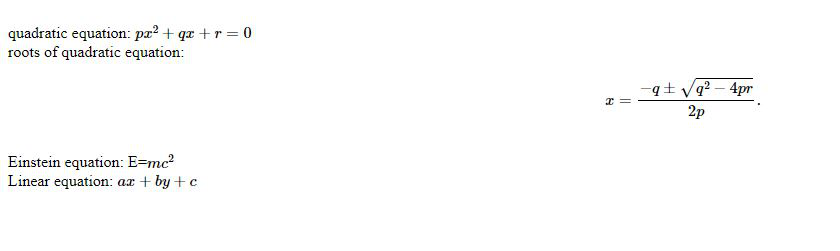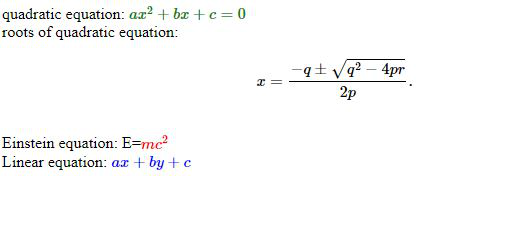# Introduction to MathJax

MathJax is a library written in JavaScript, that is used to display mathematical formulas and notations in the browsers quite easily and efficiently. It is an open-source JavaScript display engine for LaTeX, MathML, and AsciiMath notation that works in all modern browsers. MathJax does not require any kind of setup making it easier on the client-side to view it naturally and without any difficulty. This library is built on the top of MathML.

Features:

• Easy to write in the browser and flexible to read.
• Powerful API Integration.

Installation using a CDN:

<script id="MathJax-script" async
src="https://cdn.jsdelivr.net/npm/mathjax@3/es5/tex-mml-chtml.js">
</script>


Installation in Nodejs:

npm install mathjax

The below examples illustrate the use-cases of MathJax:

Example 1:

## HTML

   <html lang="en">     <head>      <meta charset="UTF-8">      <meta name="viewport" content="width=device-width,                   initial-scale=1.0">      <title>Math jax       <body>              <br />quadratic equation:      $$px^2 + qx + r = 0$$      <br />      roots of quadratic equation:      $$x = {-q \pm \sqrt{q^2-4pr} \over 2p}.$$      <br />      Einstein equation: E=$$mc^2$$      <br />      Linear equation: $$ax+by+c$$              <script id="MathJax-script" async src=  "https://cdn.jsdelivr.net/npm/mathjax@3/es5/tex-mml-chtml.js">                    <script>          MathJax = {              tex: {                  inlineMath: [['$', '$'],                               ['\$$', '\$$']]              },              svg: {                  fontCache: 'global'              }          };             

Output:Example 2: This example shows the changing of the color of the text.

## HTML

   <html lang="en">     <head>      <meta charset="UTF-8">      <meta name="viewport" content="width=device-width,                   initial-scale=1.0">      <title>Math jax       <body>                   <br />quadratic equation:      ${\color{green} ax^2+bx+c=0}$      <br />      roots of quadratic equation:      $$x = {-q \pm \sqrt{q^2-4pr} \over 2p}.$$      <br />              Einstein equation: E=${\color{red} mc^2}$      <br />      Linear equation: ${\color{blue} ax+by+c}$              <script id="MathJax-script" async src=  "https://cdn.jsdelivr.net/npm/mathjax@3/es5/tex-mml-chtml.js">                    <script>          MathJax = {              autoload: {                  color: [],                  colorV2: ['color']              },              tex: {                  inlineMath: [['$', '$'],                           ['\$$', '\$$']]              },              svg: {                  fontCache: 'global'              }          };             

Output:My Personal Notes arrow_drop_upCheck out this Author's contributed articles.

If you like GeeksforGeeks and would like to contribute, you can also write an article using contribute.geeksforgeeks.org or mail your article to contribute@geeksforgeeks.org. See your article appearing on the GeeksforGeeks main page and help other Geeks.

Please Improve this article if you find anything incorrect by clicking on the "Improve Article" button below.

Article Tags :

Be the First to upvote.

Please write to us at contribute@geeksforgeeks.org to report any issue with the above content.